# Simulate the list of STL containers

### Article directory

• foreword
• 1. General idea
• 2. Code implementation
• 1. Create node class and list class
• 2. Iterator design
• 1. Forward iterator
• 2. Reverse iterator
• 3. Other interfaces
• 1. Construction and destruction
• 2. Insertion and deletion
• 3. Summary

## Foreword

This article mainly introduces the implementation of list simulation. The essence of list simulation implementation is the implementation of iterators. By manually implementing the list, we can further understand the role of templates. There may not be many implementations of data structures in this article, and here we mainly introduce the syntax features of C++.

## 1. General idea

Before implementation, we can first look at the implementation of the list in the source code of the STL library. As early as in the blog of the data structure linked list, it was mentioned that the list in the library adopts the structure of the bidirectional circular linked list, so we also use this method to realize the list. The difficulty in the implementation of list lies in the implementation of iterators. We know that the nodes of the linked list are all new, so we cannot use native pointers as iterators. In fact, in the source code, an iterator template class is implemented in the library, through which the behavior of native pointers is simulated. Here we also use this method to implement the iterator of the list.

## 1. Create node class and list class

We know that a list is composed of nodes, so we first create a node class and a list class.`This is very different from the C language. The C language list structure has node pointer members. When C++ is designed, the node is a class alone, and the members in the list are variables of the node type. For each custom type in C++, a corresponding class is designed. In this way, it is more in line with the object-oriented design idea, and the logic of writing code will be clear. `

Code sample

```namespace Ly
{<!-- --> //Create a node template
template<class T>
struct node
{<!-- --> //Initialization for the new node later is a bit like the Buynode written before
node(const T & val = T())
:_next(nullptr)
, _prev(nullptr)
,_data(val)
{<!-- -->

}
T _data;//value domain
};

template<class T>
class list
{<!-- -->
public:
typedef node<T> node;
private:

};
}
```

The main reason why the node here is defined by struct is because for ease of access, the default permission of struct is public and will not be restricted. It is also fine to use class definition, just set the permission to public. `The default value of this parameter for anonymous objects has also been mentioned in the previous vectord blog, so I won't repeat it here. This node is given a constructor, which is for easy initialization when renewing the node. ` Here, node is renamed to node for ease of writing. The node destructor is not written, and the nodes of the linked list are finally destructed by the linked list itself, that is, the list class is destructed.

## 2. Design of iterator

### 1. Forward iterator

The most essential point of learning to realize the list is the design of the iterator. As for the addition, deletion, checking and modification of the linked list, it is all about the data structure. These logics are simple to implement, but the implementation of iterators requires us to think about it. `list cannot use native pointers as iterators, so we have to design our own generators. We can go to the stl source code to learn first, so let's just talk about it here. In the slt library, an iterator class template is written to simulate the behavior of native pointers as iterators. ` That is to say, in addition to the custom type lnode members in the list, there is also a custom type iterator. `This iterator type is the type of list iterator. `

```template<class T,class Ref,class Ptr>
struct list_iterator
{<!-- -->
typedef node<T> node;//Rename the type for easy use
typedef list_iterator self; //rename the class name for ease of use
node* _node;
list_iterator( node* new_node)
:_node(new_node)
{<!-- -->

}
self & operator ++ ()
{<!-- -->
_node=_node->_next;
return *this;
}
self operator + + (int)
{<!-- -->
self tem(_node);
_node = _node->_next;
return tem;
}
self & operator--()
{<!-- -->
_node = _node->_prev;
return *this;
}
self operator--(int)
{<!-- -->
self tem(_node);
_node = _node->_prev;
return tem;
}
Ref & operator *()
{<!-- -->
return _node->_data;
}
Ptr & operator->()
{<!-- -->
return & operator*();
}
bool operator==(const self & It)const
{<!-- -->
return _node == It._node;
}
bool operator!=(const self & It)const
{<!-- -->
return _node != It._node;
}

//The destructor does not need to be written, the list class will be destructed
};
```

The iterator here is a class template, and there are 3 template parameters. You may wonder why there are 3 template parameters? One template parameter should be enough, we will analyze this a little bit, don’t worry. Let’s analyze it from the beginning: `First of all, list cannot be a native pointer. We design a list_iterator class template to simulate a native pointer. How to simulate it? We can first let the llist_iterator class have a node type member variable, and we can use the node in the list_iterator to replace the head node heda of the linked list for traversal. As for + + -- * -> these operators, we can use operator operator overloading to simulate the behavior of native pointers, so that iterators are not implemented. `The traversal and addition, deletion, and modification of the linked list still have to visit the nodes one by one through the head pointer. The function of the iterator is to replace the head node to traverse and access the nodes. We now think of a way to make the iterator a substitute for the head node. Based on this iterator class was designed.

Because we are making iterators a replacement for head pointers, iterator classes must have member variables of type node*. Based on this, our iterator class only needs to write a parameterized constructor. `Here we rename the class name for the convenience of writing and future code changes. What should be the return value of the function overload? When we write the date class, when the operator is overloaded, it returns a date class object, then the iterator class should return an iterator class object, so this class name is our return value. `

+ + is _node=_node->next, – – is _node= _node->prve, * is _node->data. `These things are the characteristics of the two-way circular linked list, so I won't say more here. We first see this prefix ++ and prefix -- Because what is changed is itself, we return *this, and the return value is a reference. Post-- and post-post ++ should return the value before the change. Here, a temporary variable is used to save the value before ++ or --, because we return a temporary variable, so the value is returned. When we dereference, we want to get data data, so this return should be T. At the same time, when we access this data value field, we may also modify this value field, so the return value is a reference. ` But we think about a problem. In addition to ordinary iterators, we need to implement const iterators. What is the biggest difference between this const iterator and a normal iterator? That is, the value field pointed to by the const iterator cannot be modified. `It should be noted here that the value field in the node pointed to by the iterator cannot be modified, not the iterator cannot be modified. If the iterator cannot be modified, how does the iterator move through it? `So make a fuss about overloading *, if it returns T & amp; it is not an ordinary iterator, if it returns const T, it is a const iterator, based on this we introduce the second template The second template parameter of parameter Ref, ` is to instantiate the corresponding cosnt iterator and common iterator, so that common iterator and const iterator can share a set of code, and the reusability of the code is greatly enhanced. ` Let’s take a look at the image below.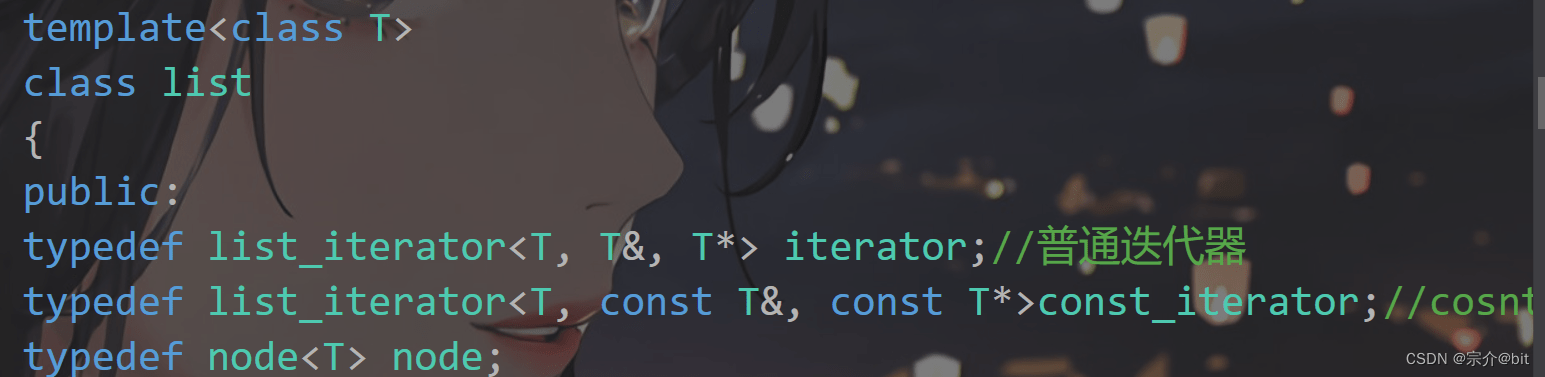`Let's ignore the third template parameter here. ` We know that the iterator is a custom type and a template class. In the list template class, the iterator class will first be instantiated into list_iterator type or list_iterator type, when the list is instantiated again, the corresponding iterator type will be instantiated again. ` This is a bit like a template within a template, so that the iterator object in the list will instantiate two different iterator members based on the same code. This way of handling is simply wonderful, and this is how it is implemented in the library. `

We know that an iterator is to simulate the pointer behavior of the corresponding object for access or traversal. If we instantiate a listlt object, we know that this Date is a custom type. If we want to use lt’s iterator to access the internal members of lt, that is, the members of the value field, then we have to overload ->. `Maybe this is too convoluted, and it is still an example of lt. If each node of the linked list is a Date, then the corresponding value field data is a Date object, then if we want to access the members of the value field Date like a pointer, just Must overload ->. ` This is the origin of the third template parameter of the iterator class.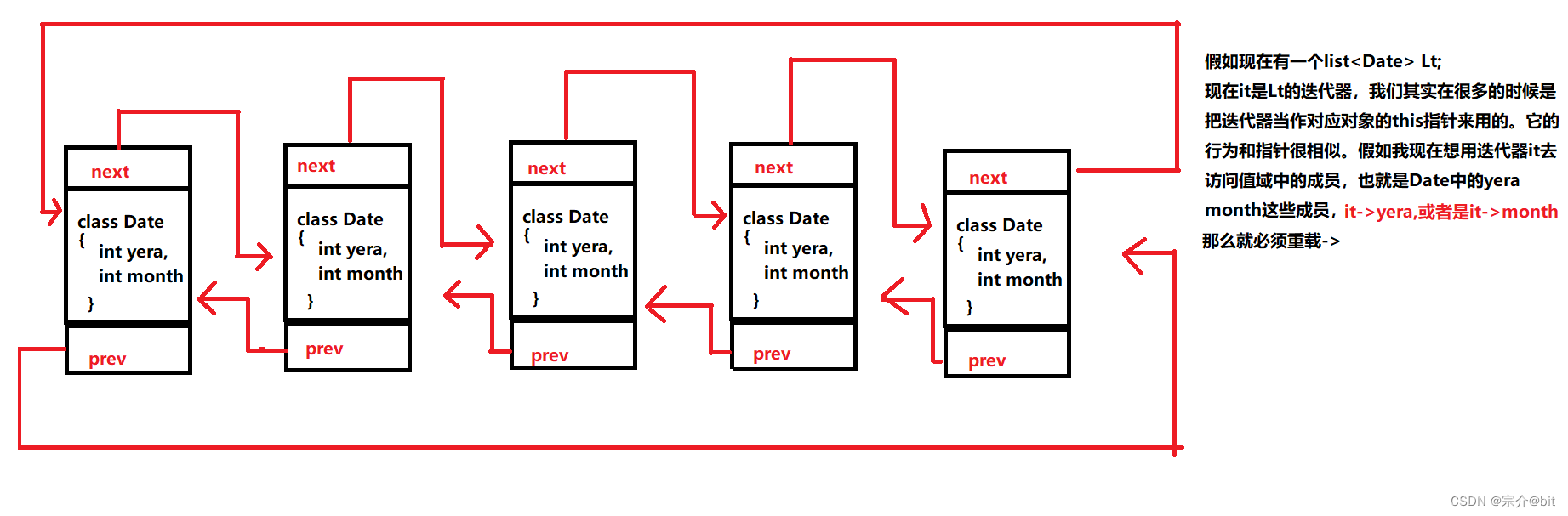```Ptr & amp; operator->()
{<!-- -->
return & operator*();
}
```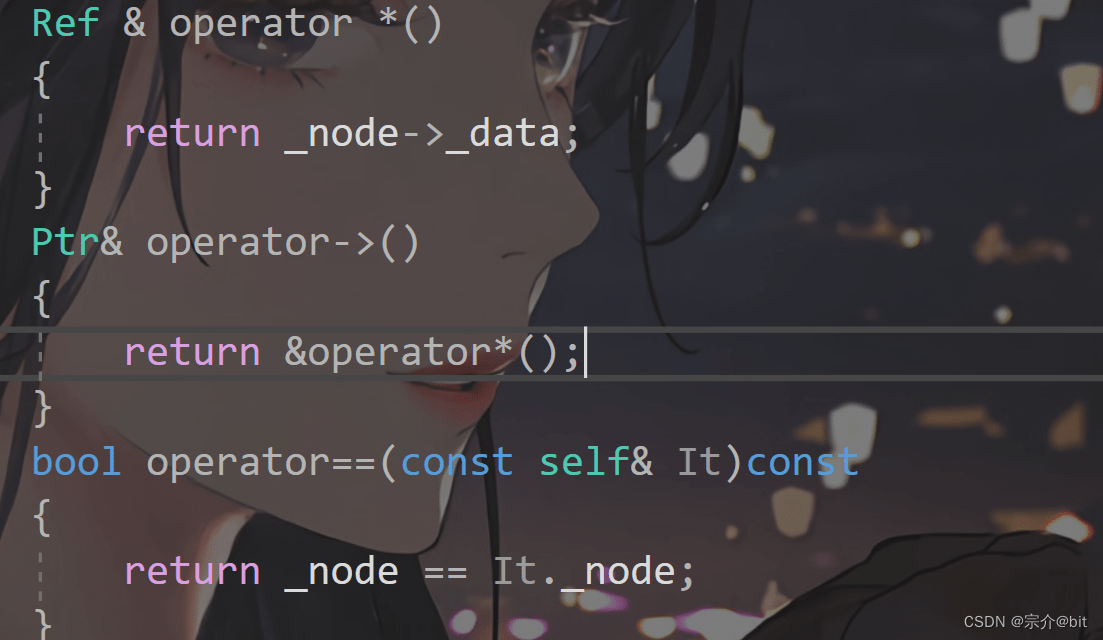`Here we see that the implementation of -> operator overloading returns &operator*(), which may be a bit confusing. But let's think about it carefully, what the * overload gets is not the corresponding value field in the node, if we take the address, it is not the this of the node value field object. We can use -> to access the member variables in the custom type. `This processing method is also very wonderful, which is also learned in the source code. Similarly, we also know the origin of the third parameter, which is to support -> operator overloading.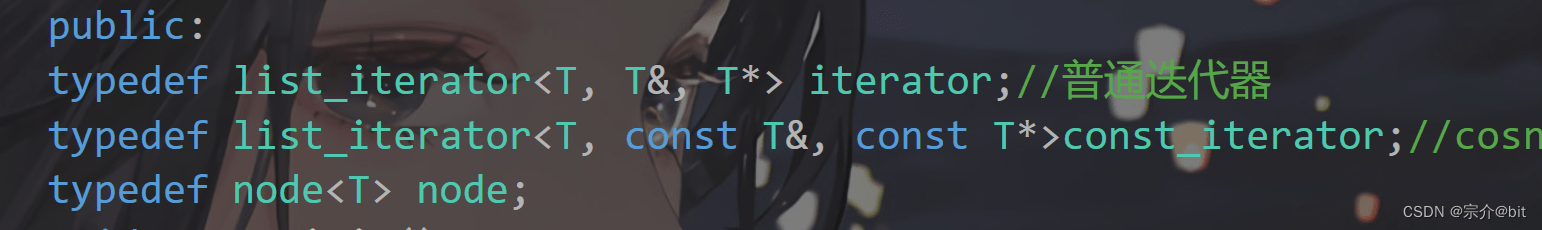Here we directly construct the iterator in the form of an anonymous object, _head->nex is the beginning of the head node, and _head is the end. This is determined by the characteristics of the doubly linked list. `The typedef here is to rename the iterator type once. Because the naming method of the iterator type is specified in the library. ` This easily solves the iterator method.

### 2. Reverse iterator

We have designed the forward iterator, so how to design the reverse iterator? We may say that it is very simple, we write a reverse iterator class according to the forward iterator, rbegin points to heda->prev rend points to head, – – + + just change the logic. According to this idea, we are very It is easy to write code with the following ideas.

```template<class T, class Ref, class Ptr>
struct __list_reverse_iterator
{<!-- -->
typedef list_node<T> node;
typedef __list_reverse_iterator<T, Ref, Ptr> self;
node* _node;

__list_reverse_iterator(node* n)
:_node(n)
{<!-- -->}

Ref operator*()
{<!-- -->
return _node->_data;
}

Ptr operator->()
{<!-- -->
return &_node->_data;
}

self & operator ++ ()
{<!-- -->
_node = _node->_prev;

return *this;
}

self operator + + (int)
{<!-- -->
self tmp(*this);
_node = _node->_prev;

return tmp;
}

self & operator--()
{<!-- -->
_node = _node->_next;

return *this;
}

self operator--(int)
{<!-- -->
self tmp(*this);
_node = _node->_next;

return tmp;
}

bool operator!=(const self & s)
{<!-- -->
return _node != s._node;
}

bool operator==(const self & s)
{<!-- -->
return_node == s._node;
}
};
```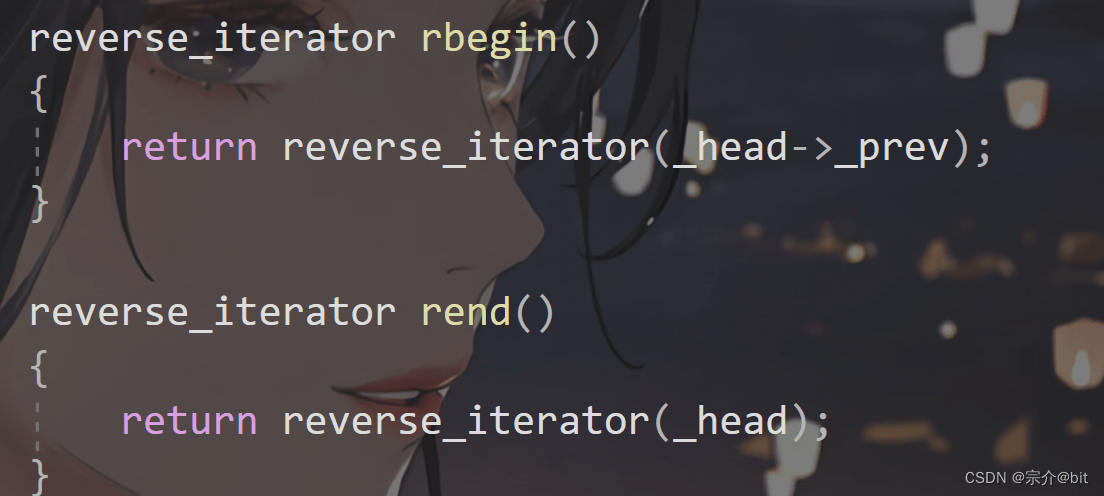Here we change the forward iterator + + to _node=_node->prev, – – to _node=_node->next. `But the library is not designed like this. The design in the library is even more wonderful. The library adapts the reverse iterator based on the forward iterator. Let's look at the code implementation in the library. `

```template<class Iterator, class Ref, class Ptr>
struct ReverseIterator
{<!-- -->
typedef ReverseIterator<Iterator, Ref, Ptr> Self;
Iterator_cur;

ReverseIterator(Iterator it)
:_cur(it)
{<!-- -->}

Ref operator*()
{<!-- -->
Iterator tmp = _cur;
--tmp;
return *tmp;
}

Self & operator ++ ()
{<!-- -->
--_cur;
return *this;
}

Self & operator--()
{<!-- -->
+ + _cur;
return *this;
}

bool operator!=(const Self & s)
{<!-- -->
return _cur != s._cur;
}
bool operator==(const Self & s)
{<!-- -->
return _cur == s._cur;
}
};
```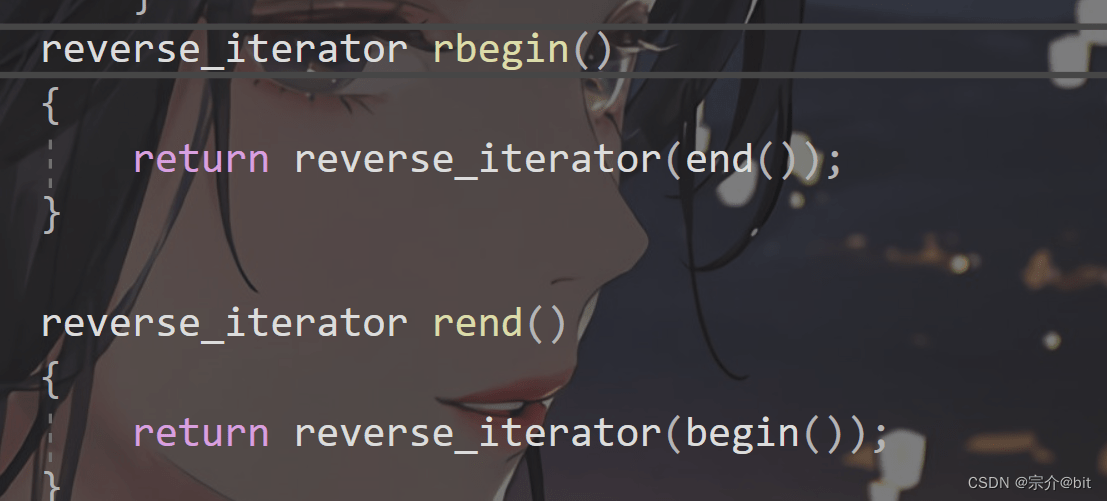Some people may have doubts about this, isn’t this still a class? Is there a difference with the above? `Let's study the code carefully, and the above reverse iterator is completely evolved according to the forward iterator. All interfaces here are actually implemented by calling the forward iterator interface. `In other words, as long as the forward iterator is implemented, the forward iterator can be adapted. `If you say this, you may not realize the benefits of this design. Let me give you an example. If I implement the forward iterator of vector now, and now I want to implement the reverse iterator, if the above method is not adopted, then I have to design a reverse iterator class and re-design a batch of interfaces. Isn't this tedious? `If the design method in the library is used, no matter what kind of container, as long as I design a pointing iterator, I can deduce a reverse iterator based on the pointing iterator. This is equivalent to reusing the code of the forward iterator, which is wonderful.

The ++ of the reverse iterator is the – – of the forward iterator, the – – of the reverse iterator is the ++ of the forward iterator, and the + + – – of the forward iterator is changed. In other words: `We change the forward iterator so that the original - - operation becomes a ++ operation, and the original ++ operation becomes a -- operation. That is to change the -- of begin end to + + + + into - -; it is the reverse iterator rbegin rend. `This is the key point of the above code. We also know that begin corresponds to the position of rend, and end corresponds to the position of rbgein. We use begin end in the list class to construct this rend and rbegin.

There are still some details here that we need to pay attention to, that is, let’s draw a picture to understand the overloaded * operator.The use of temporary variables here is very clever. Use temporary variables to dereference first. At this time, the iterator does not move. After that, the iterator goes again, so that all nodes can be visited. `The temporary variable here is the copy structure generated by default. We mainly access the node through the address of the node, so there is no problem with the copy structure generated by the compiler by default. This does not involve the application of resources. Here, because of the temporary variable used, the value is returned. Although here is an overload of the * operator that returns the forward iterator that is being called by value, that is to say, although this tem is a copy of begin or end, it will find the corresponding data value field according to the address of the node and return the value field references. The value range can still be modified like a forward iterator, because it is adapted through a forward iterator, and the interface is the set of the forward iterator that is called. `

## 3. Other interfaces

Implemented the most difficult iterator, and the rest of the interfaces are well implemented. The rest of the interface is mainly implemented according to the data structure characteristics of the linked list.

### 1. Construction and destruction

Default construction

```void empty_init()
{<!-- -->
}
//Default construction
list()
{<!-- -->
empty_init();
}
```

We wrote an empty initialization function for the default structure, which is also implemented in the library. This empty initialization is also used in subsequent constructions.

Copy construction

```void swap(const list<T> & amp; tem)
{<!-- -->
}
list(const list<T> & It)
{<!-- -->
empty_init();
list<T> tem(It.begin(),It.end());
swap(tem);
}
```

`The copy construction is the same as the previous implementation of vector, first create a temporary variable, and then exchange the head node of the temporary variable. Note here that the swap function uses reference swapping. That is to say _head is really changed. tem is constructed by an iterator, which means that this tem._head really points to a linked list. This implements copy construction. `

Iterator construction

```template<class Iterator>
list(Iterator frist, Iterator last)
{<!-- -->
empty_init();
while (first != last)
{<!-- -->
push_back(*frist);
+ + frist;
}
}
```

We first initialize and create a head node and then call the tail insertion interface. When the tail insertion is performed, the node will be created and the code can be reused.

Destructor

```~list()
{<!-- -->
clear();
}
void clear()
{<!-- -->
iterator it = begin();
while (it != end())
{<!-- -->
//This it is the post-value ++, and the return is the value after it ++, but it has not changed
// Don't worry about iterator invalidation
erase(it++);
}
}
```

This clear function is to delete the chain until there is only one head node. Here is the multiplexed erase for deletion. `Here is the front ++ delete, that is, the value before ++ is returned, but it has moved forward. In this way, there is no need to consider the problem of each iterator invalidation. `

### 2. Insertion and deletion

```void insert(iterator pos, const T & amp; val)
{<!-- -->
node*cur = pos._node;
node* prev = cur->_prev;
node* new_node = new node(val);
new_node->_next = cur;
new_node->_prev = prev;
cur->_prev = new_node;
prev->_next = new_node;
\t\t
}
iterator erase(iterator pos)
{<!-- -->
assert(pos != end());
node* cur = pos._node;
node* next = pos._node->_next;
node* prev = cur->_prev;
prev->_next = next;
next->_prev = prev;
delete cur;
return iterator(next);

}
```

Insert before the pos position inserted here, and delete at the specified position. The deletion and insertion logic will not be explained too much here. `What should be considered here is the problem of iterator failure, because the insertion is inserted before pos, that is to say, which node pos pointed to before is still pointing to which node now. The only thing that changes is the relative position in the linked list. For example, pos originally pointed to the third node of the linked list. After inserting a new node, it is the fourth node of the linked list. The insertion here will not cause the iterator invalidation problem. ` So will deletion cause iterator invalidation? `The nodes pointed to by pos are all released, and the iterator will definitely fail, so we have a return value. Returns the node pointed to by the next of the deleted node. `It should be noted here that the head node must not be deleted.

```void push_back(const T & amp; val)
{<!-- -->
insert(end(),val);
}
void push_front(const T & val)
{<!-- -->
insert(begin(), val);
}
void pop_back()
{<!-- -->
erase(--end());
}
void pop_front()
{<!-- -->
erase(begin());
}
```

Here, head-to-tail insertion and deletion directly reuse insertion and deletion, but attention should be paid to the position. `We see this tail insertion, the insertion is inserted before pos, and the prev of end points to the tail node. Deletion is to delete at the specified position, and the prev of end is the tail, so --end is the tail node. `

## 3. Summary

The focus of the list simulation implementation is the design of the iterator. The forward iterator is implemented first, and the reverse iterator is derived from the forward iterator. I didn’t say much about const forward iterators and const reverse iterators, because const iterators are not very simple with ordinary iterators. Pay attention to renaming the const iterator type in the list. These iterator types are all instantiated according to the iterator class. Then instantiate it again according to the list template parameter. If there is any problem with the above content, please correct me!